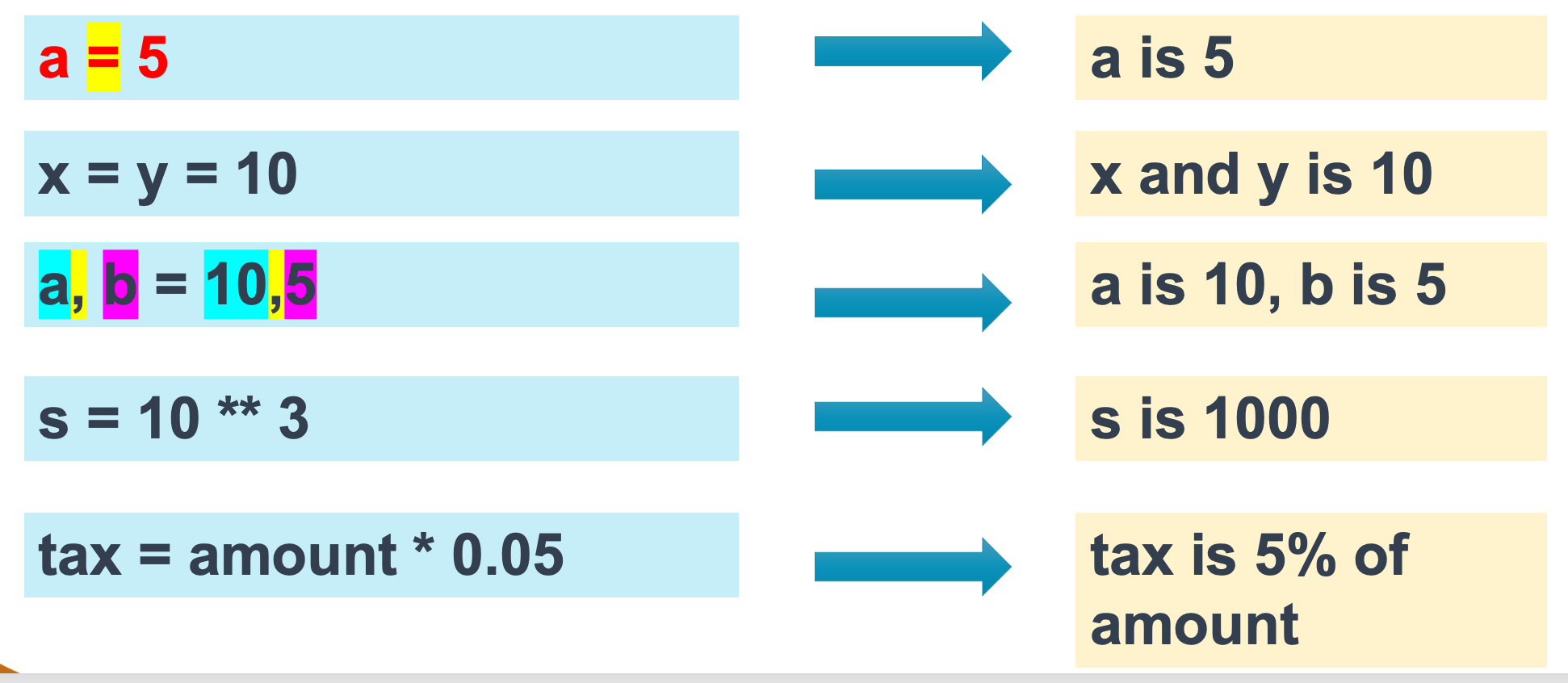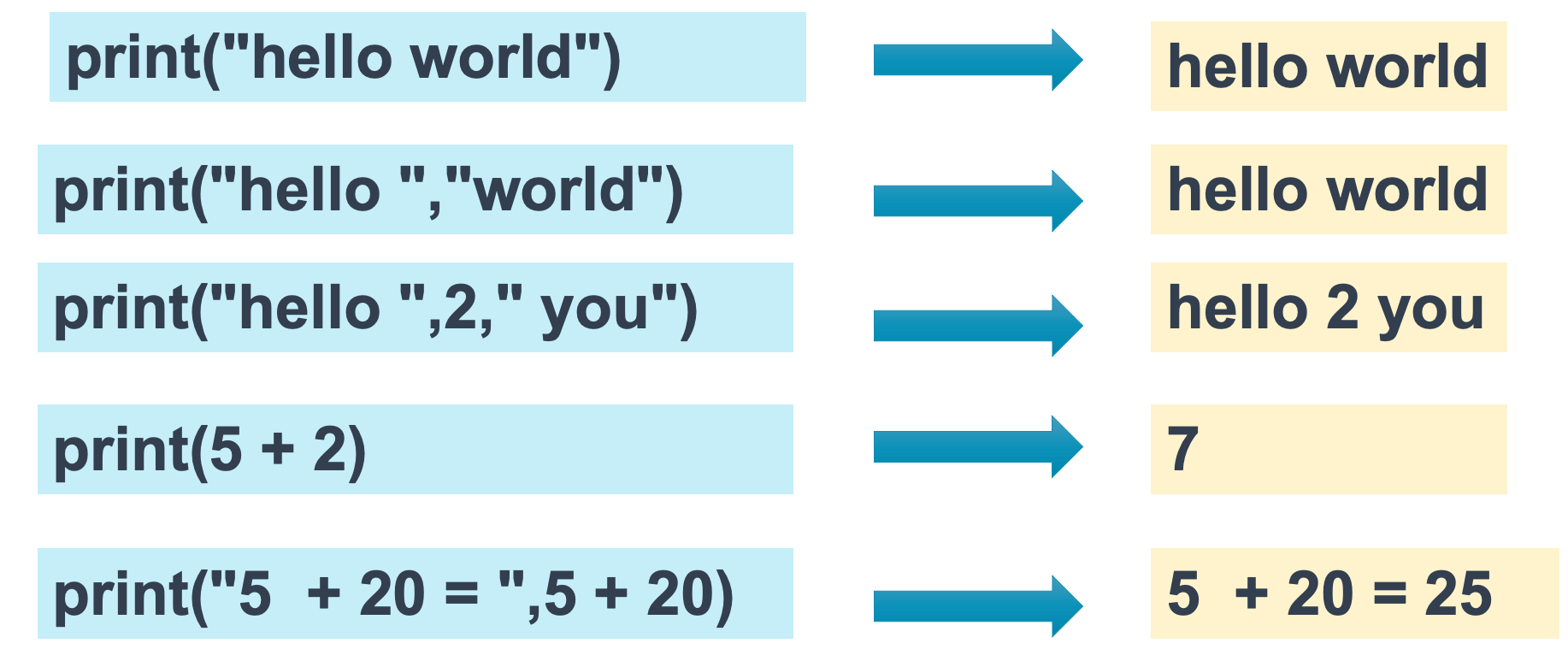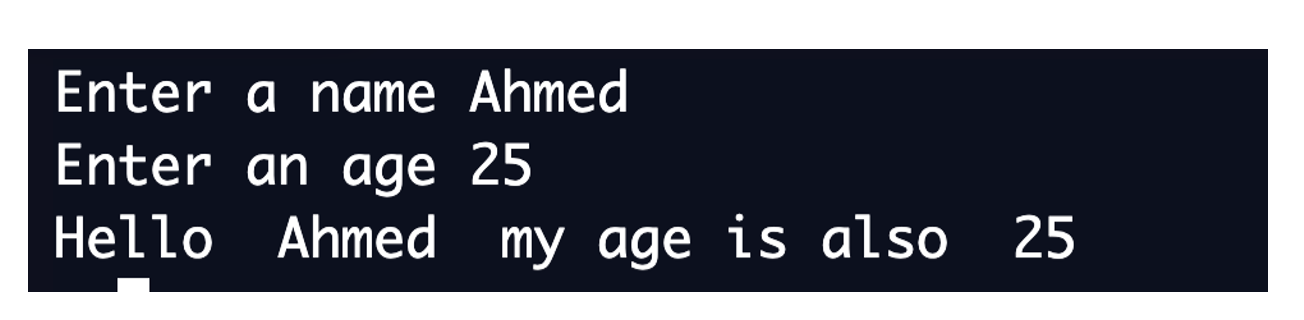# Input, Process & Output

## Assignment

variable = expression
The = operator assign the expression on the right side to the variable in left side.## Interaction with user

To read/get a value from the user:
• use the command: input
To display a value to the user:
• Use the command: print
• On-screen display

## Display statement

print(expression[,expression2, expression3,…])
The print() function prints the given expression on the console screenvariable = input(string)

• Any entry is considered as a string
• Must Convert the entry to the desired data type

### Example: ask the user for his name and his age

```	    name = input("Enter your name:")
age = int(age)
```## Input convertion

x = input("Enter anything:")

• The data type of x is string
• To convert the x into int: x = int(x)
• To Convert the x into float: x = float(x)

### Example: Program to read name and age from user and display it

Input
```	    name = input("Enter a name")
age = input("Enter an age ")
```
Output
```	    print("Hello ",name," my age is also ",age)
```

Here is the output:### Example-2: with simple calculation

Program to read name and age from user and calculate and display his/her age on next year.
```	    name = input("Enter a name”)
age = input("Enter an age ”)
age = int(age)
ageNextYear = age + 1
print("Hello ",name," you will be ", ageNextYear, "next year" )
```

### Example-3: with simple calculation

Write a Python program that read price of an item and quantity bought, calculate and display the total.
```	    price = input("Enter the price of item ")
price = float(price)
qty = input("Enter the quantity ")
qty = int(qty
total = price * qty
print("Total price ",total," dhs")
```
Here is the output: